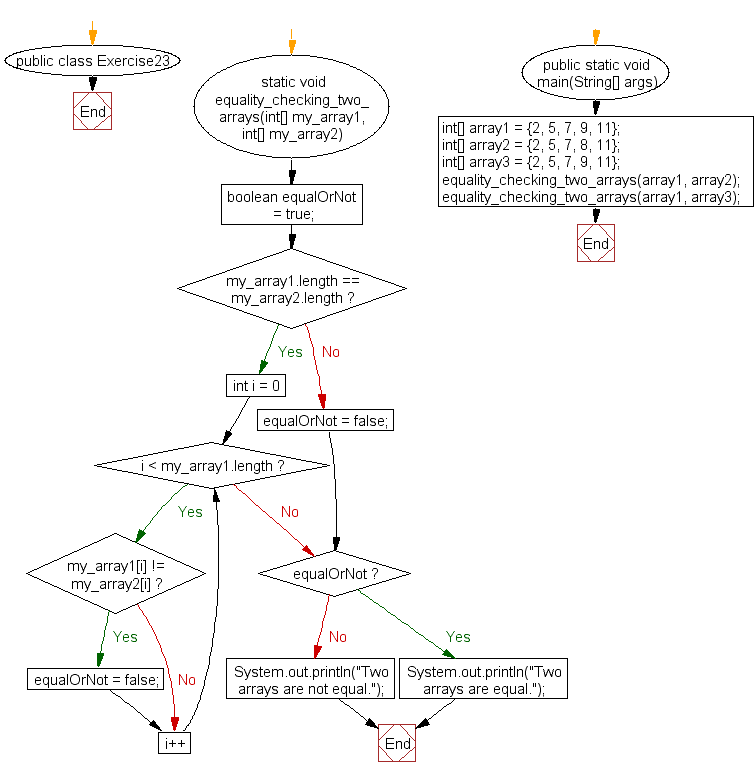﻿ Java exercises: Test the equality of two arrays - w3resource# Java Array Exercises: Test the equality of two arrays

## Java Array: Exercise-23 with Solution

Write a Java program to test the equality of two arrays.

Pictorial Presentation:Sample Solution:

Java Code :

``````public class Exercise23 {
static void  equality_checking_two_arrays(int[] my_array1, int[] my_array2)
{
boolean  equalOrNot = true;

if(my_array1.length == my_array2.length)
{
for (int i  = 0; i < my_array1.length; i++)
{
if(my_array1[i] != my_array2[i])
{
equalOrNot = false;
}
}
}
else
{
equalOrNot  = false;
}

if  (equalOrNot)
{
System.out.println("Two arrays are equal.");
}
else
{
System.out.println("Two  arrays are not equal.");
}
}

public static void  main(String[] args)
{
int[] array1 =  {2, 5, 7, 9, 11};
int[] array2 =  {2, 5, 7, 8, 11};
int[] array3 =  {2, 5, 7, 9, 11};

equality_checking_two_arrays(array1,  array2);
equality_checking_two_arrays(array1, array3);
}
}
```
```

Sample Output:

```Two arrays are not equal.
Two arrays are equal.
```

Flowchart:Visualize Java code execution (Python Tutor):

Java Code Editor:

Improve this sample solution and post your code through Disqus

What is the difficulty level of this exercise?

Test your Programming skills with w3resource's quiz.

﻿

## Java: Tips of the Day

getEnumMap

Converts to enum to Map where key is the name and value is Enum itself.

```public static <E extends Enum<E>> Map<String, E> getEnumMap(final Class<E> enumClass) {
return Arrays.stream(enumClass.getEnumConstants())
.collect(Collectors.toMap(Enum::name, Function.identity()));
}
```

Ref: https://bit.ly/3xXcFZt